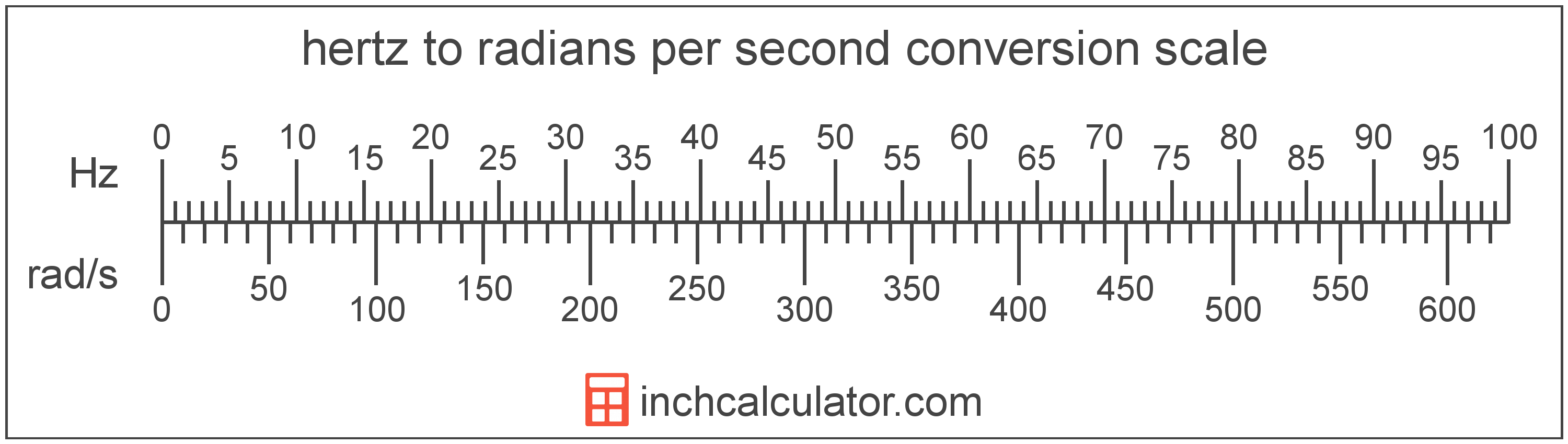# Hertz to Radians per Second Converter

Enter the frequency in hertz below to get the value converted to radians per second.Do you want to convert radians per second to hertz?

## How to Convert Hertz to Radians per Second

To convert a measurement in hertz to a measurement in radians per second, multiply the frequency by the following conversion ratio: 6.283185 radians per second/hertz.

Since one hertz is equal to 6.283185 radians per second, you can use this simple formula to convert:

radians per second = hertz × 6.283185

The frequency in radians per second is equal to the frequency in hertz multiplied by 6.283185.

For example, here's how to convert 5 hertz to radians per second using the formula above.### How Many Radians per Second Are in a Hertz?

There are 6.283185 radians per second in a hertz, which is why we use this value in the formula above.

The following formula can also be used to convert the angular frequency in radians per second to frequency in hertz:

ω = 2πƒ

In other words, the angular frequency ω in radians per second is equal to 2 times pi times the frequency ƒ in hertz.

## What is a Hertz?

One hertz is formally defined as the frequency of one cycle per second.

The hertz is the SI derived unit for frequency in the metric system. Hertz can be abbreviated as Hz; for example, 1 hertz can be written as 1 Hz.

Frequency in hertz can be expressed using the formula:

Hz = Cycles / Time in Seconds

## What Are Radians per Second?

Radians per second are a measure of angular frequency, or rotational speed, equal to the change in the angular orientation of an object over one second.

In the expressions of units, the slash, or solidus (/), is used to express a change in one or more units relative to a change in one or more other units. For example, rad/s is expressing a change in angle relative to a change in time.

## Hertz to Radian per Second Conversion Table

Table showing various hertz measurements converted to radians per second.